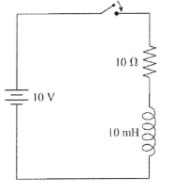# Problem: Consider the LR circuit shown belowa. What is the current through the resistor immediately after the switch closes?b. What is the battery current through the resistor after the switch has been closed a long time?

###### FREE Expert Solution

Current in an LR circuit:

$\overline{){\mathbf{i}}{\mathbf{=}}{{\mathbf{i}}}_{{\mathbf{0}}}{\mathbf{\left(}}{\mathbf{1}}{\mathbf{-}}{{\mathbf{e}}}^{\mathbf{-}\mathbf{t}}{\mathbf{\lambda }}}{\mathbf{\right)}}}$

a.

When t = 0s, i = 0 A###### Problem Details

Consider the LR circuit shown below

a. What is the current through the resistor immediately after the switch closes?

b. What is the battery current through the resistor after the switch has been closed a long time?